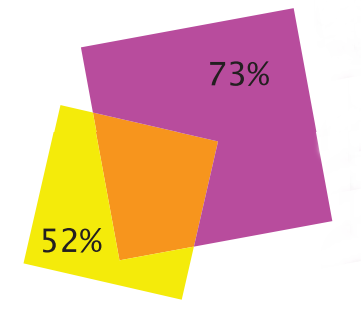# Middle man

Geometry Level 2Diagram above shows two pieces of overlapping paper. The non-overlapping area of the smaller square corresponds to 52% of the area of this square and non-overlapping area of the larger square is 73% of the area of this square. What is the ratio between the sides of the smaller square and the largest square?

×